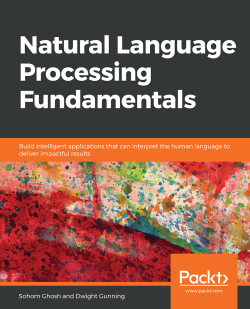### Natural Language Processing Fundamentals5 (1 reviews total)
By Sohom Ghosh , Dwight Gunning
• 7-day trial Subscribe Access now
• \$39.99 Print
• \$27.99 eBook

• Constantly updated with 100+ new titles each month
• Breadth and depth in over 1,000+ technologies

If NLP hasn't been your forte, Natural Language Processing Fundamentals will make sure you set off to a steady start. This comprehensive guide will show you how to effectively use Python libraries and NLP concepts to solve various problems.

You'll be introduced to natural language processing and its applications through examples and exercises. This will be followed by an introduction to the initial stages of solving a problem, which includes problem definition, getting text data, and preparing it for modeling. With exposure to concepts like advanced natural language processing algorithms and visualization techniques, you'll learn how to create applications that can extract information from unstructured data and present it as impactful visuals. Although you will continue to learn NLP-based techniques, the focus will gradually shift to developing useful applications. In these sections, you'll understand how to apply NLP techniques to answer questions as can be used in chatbots.

By the end of this book, you'll be able to accomplish a varied range of assignments ranging from identifying the most suitable type of NLP task for solving a problem to using a tool like spacy or gensim for performing sentiment analysis. The book will easily equip you with the knowledge you need to build applications that interpret human language.

Publication date:
March 2019
Publisher
Packt
Pages
374
ISBN
9781789954043

# 1. Introduction to Natural Language Processing

## Learning Objectives

By the end of this chapter, you will be able to:

• Describe what natural language processing (NLP) is all about
• Describe the history of NLP
• Differentiate between NLP and Text Analytics
• Describe the various phases of an NLP project

In this chapter, you will learn about the basics of natural language processing and various preprocessing steps that are required to clean and analyze the data.

# Introduction

To start with looking at NLP, let's understand what natural language is. In simple terms, it's the language we use to express ourselves. It's a basic means of communication. To define more specifically, language is a mutually agreed set of protocols involving words/sounds we use to communicate with each other.

In this era of digitization and computation, we tend to comprehend language scientifically. This is because we are constantly trying to make inanimate objects understand us. Thus, it has become essential to develop mechanisms by which language can be fed to inanimate objects such as computers. NLP helps us do this.

Let's look at an example. You must have some emails in your mailbox that have been automatically labeled as spam. This is done with the help of NLP. Here, an inanimate object – the email service – analyzes the content of the emails, comprehends it, and then further decides whether these emails need to be marked as spam or not.

# History of NLP

NLP is an area that overlaps with others. It has emerged from fields such as artificial intelligence, linguistics, formal languages, and compilers. With the advancement of computing technologies and the increased availability of data, the way natural language is being processed has changed. Previously, a traditional rule-based system was used for computations. Today, computations on natural language are being done using machine learning and deep learning techniques.

The major work on machine learning-based NLP started during the 1980s. During the 1980s, developments across various disciplines such as artificial intelligence, linguistics, formal languages, and computations led to the emergence of an interdisciplinary subject called NLP. In the next section, we'll look at text analytics and how it differs from NLP.

# Text Analytics and NLP

Text analytics is the method of extracting meaningful insights and answering questions from text data. This text data need not be a human language. Let's understand this with an example. Suppose you have a text file that contains your outgoing phone calls and SMS log data in the following format: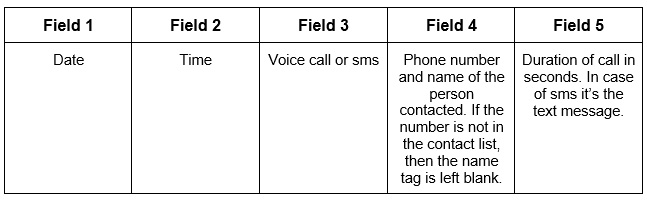##### Figure 1.1: Format of call data

In the preceding figure, the first two fields represent the date and time at which the call was made or the SMS was sent. The third field represents the type of data. If the data is of the call type, then the value for this field will be set as voice_call. If the type of data is sms, the value of this field will be set to sms. The fourth field is for the phone number and name of the contact. If the number of the person is not in the contact list, then the name value will be left blank. The last field is for the duration of the call or text message. If the type of the data is voice_call, then the value in this field will be the duration of that call. If the type of data is sms, then the value in this field will be the text message.

The following figure shows records of call data stored in a text file: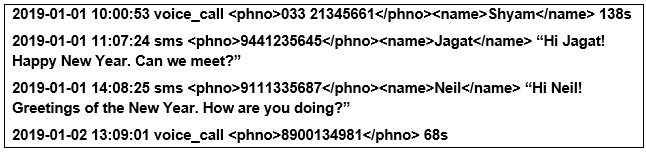##### Figure 1.2: Call records in a text file

Now, the data shown in the preceding figure is not exactly a human language. But it contains various information that can be extracted by analyzing it. A couple of questions that can be answered by looking at this data are as follows:

• How many New Year greetings were sent by SMS on 1st January?
• How many people were contacted whose name is not in the contact list?

The art of extracting useful insights from any given text data can be referred to as text analytics. NLP, on the other hand, is not just restricted to text data. Voice (speech) recognition and analysis also come under the domain of NLP. NLP can be broadly categorized into two types: Natural Language Understanding (NLU) and Natural Language Generation (NLG). A proper explanation of these terms is provided as follows:

• NLU: NLU refers to a process by which an inanimate object with computing power is able to comprehend spoken language.
• NLG: NLG refers to a process by which an inanimate object with computing power is able to manifest its thoughts in a language that humans are able to understand.

For example, when a human speaks to a machine, the machine interprets the human language with the help of the NLU process. Also, by using the NLG process, the machine generates an appropriate response and shares that with the human, thus making it easier for humans to understand. These tasks, which are part of NLP, are not part of text analytics. Now we will look at an exercise that will give us a better understanding of text analytics.

## Exercise 1: Basic Text Analytics

In this exercise, we will perform some basic text analytics on the given text data. Follow these steps to implement this exercise:

1. Open a Jupyter notebook.
2. Insert a new cell. Assign a `sentence` variable with '`The quick brown fox jumps over the lazy dog`'. Insert a new cell and add the following code to implement this:
`sentence = 'The quick brown fox jumps over the lazy dog'`
3. Check whether the word '`quick`' belongs to that text using the following code:
`'quick' in sentence`

The preceding code will return the output 'True'.

4. Find out the `index` value of the word '`fox`' using the following code:
`sentence.index('fox')`

The code will return the output 16.

5. To find out the rank of the word '`lazy`', use the following code:
`sentence.split().index('lazy')`

The code generates the output 7.

6. For printing the third word of the given text, use the following code:
`sentence.split()`

This will return the output 'brown'.

7. To print the third word of the given sentence in reverse order, use the following code:
`sentence.split()[::-1]`

This will return the output 'nworb'.

8. To concatenate the first and last words of the given sentence, use the following code:
`words = sentence.split()first_word = wordslast_word = words[len(words)-1]concat_word = first_word + last_word print(concat_word)`

The code will generate the output 'Thedog'.

9. For printing words at even positions, use the following code:
`[words[i] for i in range(len(words)) if i%2 == 0]`

The code generates the following output: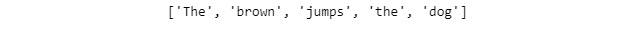##### Figure 1.3: List of words at even positions
10. To print the last three letters of the text, use the following code:
`sentence[-3:]`

This will generate the output 'dog'.

11. To print the text in reverse order, use the following code:
`sentence[::-1]`

The code generates the following output: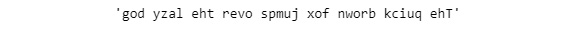##### Figure 1.4: Text in reverse order
12. To print each word of the given text in reverse order, maintaining their sequence, use the following code:
`print(' '.join([word[::-1] for word in words]))`

The code generates the following output: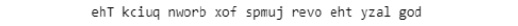##### Figure 1.5: Printing the text in reverse order while preserving word sequence

We are now well acquainted with NLP. In the next section, let's dive deeper into the various steps involved in it.

# Various Steps in NLP

Earlier, we talked about the types of computations that are done on natural language. There are various standard NLP tasks. Apart from these tasks, you have the ability to design your own tasks as per your requirements. In the coming sections, we will be discussing various preprocessing tasks in detail and demonstrating them with an exercise.

## Tokenization

Tokenization refers to the procedure of splitting a sentence into its constituent words. For example, consider this sentence: "I am reading a book." Here, our task is to extract words/tokens from this sentence. After passing this sentence to a tokenization program, the extracted words/tokens would be "I", "am", "reading", "a", "book", and ".". This example extracts one token at a time. Such tokens are called unigrams. However, we can also extract two or three tokens at a time. We need to extract tokens because, for the sake of convenience, we tend to analyze natural language word by word. If we extract two tokens at a time, it is called bigrams. If three tokens, it is called trigrams. Based on the requirements, n-grams can be extracted (where "n" is a natural number).

### Note

n-gram refers to a sequence of n items from a given text.

Let's now try extracting trigrams from the following sentence: "The sky is blue." Here, the first trigram would be "The sky is". The second would be "sky is blue". This might sound easy. However, tokenization can be difficult at times. For instance, consider this sentence: "I would love to visit the United States". The tokens generated are "I", "would", "love", "to", "visit", "the", and "United States". Here, "United States" has to be treated as a single entity. Individual words such as "United" and "States" do not make any sense here.

To get a better understanding of tokenization, let's solve an exercise based on it in the next section.

## Exercise 2: Tokenization of a Simple Sentence

In this exercise, we will tokenize the words in a given sentence with the help of the NLTK library. Follow these steps to implement this exercise:

1. Open a Jupyter notebook.
2. Insert a new cell and add a following code to import the necessary libraries:
```import nltk
from nltk import word_tokenize```
3. The `word_tokenize()` method is used to split the sentence into words/tokens. We need to add a sentence as input to the `word_tokenize()` method, so that it performs its job. The result obtained would be a list, which we will store in a `word` variable. To implement this, insert a new cell and add the following code:
`words = word_tokenize("I am reading NLP Fundamentals")`
4. In order to view the list of tokens generated, we need to view it using the `print()` function. Insert a new cell and add the following code to implement this:
`print(words)`

The code generates the following output: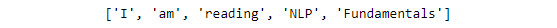##### Figure 1.6: List of tokens

Thus we can see the list of tokens generated with the help of the `word_tokenize()` method. In the next section, we will see another pre-processing step: Parts-of-Speech (PoS) tagging.

## PoS Tagging

PoS refers to parts of speech. PoS tagging refers to the process of tagging words within sentences into their respective parts of speech and then finally labeling them. We extract Part of Speech of tokens constituting a sentence, so that we can filter out the PoS that are of interest and analyze them. For example, if we look at the sentence, "The sky is blue," we get four tokens – "The," "sky," "is," and "blue" – with the help of tokenization. Now, using PoS tagger, we tag parts of speech to each word/token. This will look as follows:

[('The', 'DT'), ('sky', 'NN'), ('is', 'VBZ'), ('blue', 'JJ')]

DT = determiner

NN = noun, common, singular or mass

VBZ = verb, present tense, 3rd person singular

An exercise in the next section will definitely give a better understanding of this concept.

## Exercise 3: PoS Tagging

In this exercise, we will find out the PoS for each word in the sentence, "`I am reading NLP Fundamentals`". We first make use of tokenization in order to get the tokens. Later, we use a PoS tagger, which will help us find PoS for each word/token. Follow these steps to implement this exercise:

1. Open a Jupyter notebook.
2. Insert a new cell and add the following code to import the necessary libraries:
```import nltk
from nltk import word_tokenize```
3. For finding the tokens in the sentence, we make use of the `word_tokenize()` method. Insert a new cell and add the following code to implement this:
`words = word_tokenize("I am reading NLP Fundamentals")`
4. Print the tokens with the help of the `print()` function. To implement this, add a new cell and write the following code:
`print(words)`

The code generates the following output: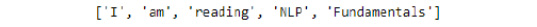##### Figure 1.7: List of tokens
5. In order to find the PoS for each word, we make use of the `pos_tag()` method of the `nltk` library. Insert a new cell and add the following code to implement this:
`nltk.pos_tag(words)`

The code generates the following output:##### Figure 1.8: PoS tag of words
6. In the preceding output, we can see that for each token, a PoS has been allotted. Here, PRP stands for personal pronoun, VBP stands for verb present, VGB stands for verb gerund, NNP stands for proper noun singular, and NNS stands for noun plural.

We have learned about labeling appropriate PoS to tokens in a sentence. In the next section, we will learn about stop words in sentences and ways to deal with them.

## Stop Word Removal

Stop words are common words that are just used to support the construction of sentences. We remove stop words from our analysis as they do not impact the meaning of sentences they are present in. Examples of stop words include a, am, and the. Since they occur very frequently and their presence doesn't have much impact on the sense of the sentence, they need to be removed.

In the next section, we will look at the practical implementation of removing stop words from a given sentence.

## Exercise 4: Stop Word Removal

In this exercise, we will check the list of stopwords provided by the `nltk` library. Based on this list, we will filter out the stopwords included in our text. Follow these steps to implement this exercise:

1. Open a Jupyter notebook.
2. Insert a new cell and add the following code to import the necessary libraries:
```import nltk
from nltk import word_tokenize
from nltk.corpus import stopwords```
3. In order to check the list of stopwords provided for the `English` language, we pass it as a parameter to the `words()` function. Insert a new cell and add the following code to implement this:
`stop_words = stopwords.words('English')`
4. In the code, the list of stopwords provided by the `English` language is stored in the `stop_words` variable. In order to view the list, we make use of the `print()` function. Insert a new cell and add the following code to view the list:
`print(stop_words)`

The code generates the following output: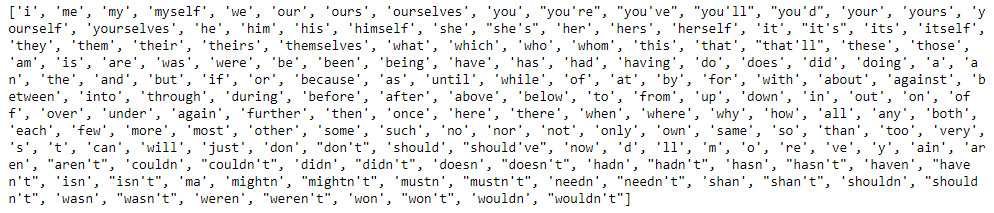##### Figure 1.9: List of stopwords provided by the English language
5. To remove the stop words from a sentence, we first assign a string to the `sentence` variable and tokenize it into words using the `word_tokenize()` method. Insert a new cell and add the following code to implement this:
```sentence = "I am learning Python. It is one of the most popular programming languages"
sentence_words = word_tokenize(sentence)```
6. To print the list of tokens, insert a new cell and add the following code:
`print(sentence_words)`

The code generates the following output: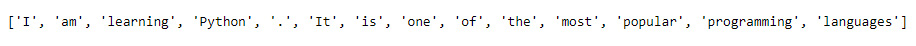##### Figure 1.10: List of tokens in the sentence_words variable
7. To remove the stopwords, first we need to loop through each word in the sentence, check whether there are any stop words, and then finally combine them to form a complete sentence. To implement this, insert a new cell and add the following code:
`sentence_no_stops = ' '.join([word for word in sentence_words if word not in stop_words])`
8. To check whether the stopwords are filtered out from our sentence, we print the `sentence_no_stops` variable. Insert a new cell and add the following code to print:
`print(sentence_no_stops)`

The code generates the following output: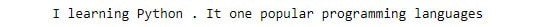##### Figure 1.11: Text without stopwords

As you can see in the preceding figure, stopwords such as "am," "is," "of," "the," and "most" are being filtered out and text without stop words is produced as output.

We have learned how to remove stop words from given text. In the next section, we will focus on normalizing text.

## Text Normalization

There are some words that are spelt, pronounced, and represented differently, for example, words such as Mumbai and Bombay, and US and United States. Although they are different, they mean the same thing. There are also different forms words that need to be converted into base forms. For example, words such as "does" and "doing," when converted to their base form, become "do". Along these lines, text normalization is a process wherein different variations of text get converted into a standard form. We need to perform text normalization as there are some words that can mean the same thing as each other. There are various ways of normalizing text, such as spelling correction, stemming, and lemmatization, which will be covered later.

For a better understanding of this topic, we will look into practical implementation in the next section.

## Exercise 5: Text Normalization

In this exercise, we will normalize a given text. Basically, we will be trying to replace selected words with new words, using the `replace()` function, and finally produce the normalized text. Follow these steps to implement this exercise:

1. Open a Jupyter notebook.
2. Insert a new cell and add the following code to assign a string to the `sentence` variable:
`sentence = "I visited US from UK on 22-10-18"`
3. We want to replace "`US`" with "`United States`", "`UK`" with "`United Kingdom`", and "`18`" with "`2018`". To do so, we make use of the `replace()` function and store the updated output in the "`normalized_sentence`" variable. Insert a new cell and add the following code to implement this:
`normalized_sentence = sentence.replace("US", "United States").replace("UK", "United Kingdom").replace("-18", "-2018")`
4. Now, in order to check whether the text has been normalized, we insert a new cell and add the following code to print it:
`print(normalized_sentence)`

The code generates the following output: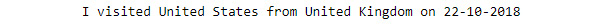##### Figure 1.12: Normalized text

In the preceding figure, we can see that our text has been normalized.

Now that we have learned the basics of text normalization, in the next section, we explore various other ways that text can be normalized.

## Spelling Correction

Spelling correction is one of the most important tasks in any NLP project. It can be time consuming, but without it there are high chances of losing out on required information. We make use of the "autocorrect" Python library to correct spellings. Let's look at the following exercise to get a better understanding of this.

## Exercise 6: Spelling Correction of a Word and a Sentence

In this exercise, we will perform spelling correction on a word and a sentence, with the help of the '`autocorrect`' library of Python. Follow these steps in order to implement this exercise:

1. Open a Jupyter notebook.
2. Insert a new cell and add the following code to import the necessary libraries:
```import nltk
from nltk import word_tokenize
from autocorrect import spell```
3. In order to correct the spelling of a word, pass a wrongly spelled word as a parameter to the `spell()` function. Insert a new cell and add the following code to implement this:
`spell('Natureal')`

The code generates the following output: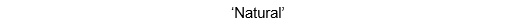##### Figure 1.13: Corrected word
4. In order to correct the spelling of a sentence, we first need to tokenize it into words. After that, we loop through each word in `sentence`, autocorrect them, and finally combine them. Insert a new cell and add the following code to implement this:
`sentence = word_tokenize("Ntural Luanguage Processin deals with the art of extracting insightes from Natural Languaes")`
5. We make use of the `print()` function to print all tokens. Insert a new cell and add the following code to print tokens:
`print(sentence)`

The code generates the following output: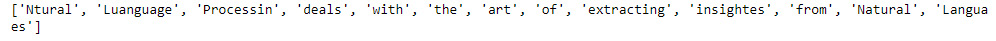##### Figure 1.14: Tokens in sentences
6. Now that we have got the tokens, we loop through each token in `sentence`, correct them, and assign them to new variable. Insert a new cell and add the following code to implement this:
`sentence_corrected = ' '.join([spell(word) for word in sentence])`
7. To print the correct sentence, we insert a new cell and add the following code:
`print(sentence_corrected)`

The code generates the following output: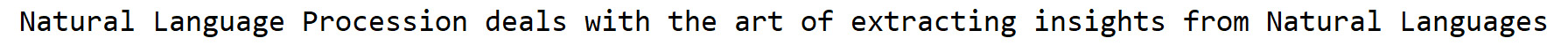##### Figure 1.15: Corrected sentence
8. In the preceding figure, we can see that most of the wrongly spelled words have been corrected. But the word "Processin" was wrongly converted into "Procession". It should have been "Processing". It happened because to change "Processin" to "Procession" or "Processing," an equal number of edits is required. To rectify this, we need to use other kinds of spelling correctors that are aware of context.

In the next section, we will look at stemming, which is another form of text normalization.

## Stemming

In languages such as English, words get transformed into various forms when being used in a sentence. For example, the word "product" might get transformed into "production" when referring to the process of making something or transformed into "products" in plural form. It is necessary to convert these words into their base forms, as they carry the same meaning. Stemming is a process that helps us in doing so. If we look at the following figure, we get a perfect idea about how words get transformed into their base forms:##### Figure 1.16: Stemming of the word product

To get a better understanding about stemming, we shall look into an exercise in the next section.

## Exercise 7: Stemming

In this exercise, we will pass a few words through the stemming process such that they get converted into their base forms. Follow these steps to implement this exercise:

1. Open a Jupyter notebook.
2. Insert a new cell and add the following code to import the necessary libraries:
```import nltk
stemmer = nltk.stem.PorterStemmer()```
3. Now pass the following words as parameters to the `stem()` method. To implement this, insert a new cell and add the following code:
`stemmer.stem("production")`

When the input is "`production`", the following output is generated: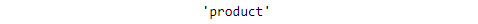##### Figure 1.17: Stemmed word for production
`stemmer.stem("coming")`

When the input is "`coming`", the following output is generated: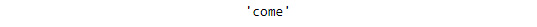##### Figure 1.18: Stemmed word for coming
`stemmer.stem("firing")`

When the input is "`firing`", the following output is generated: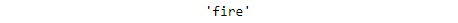##### Figure 1.19: Stemmed word for firing
`stemmer.stem("battling")`

When the input is "`battling`", the following output is generated: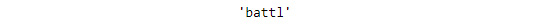##### Figure 1.20: Stemmed word for battling
4. From the preceding figures, we can see that the entered words are converted into their base forms.

In the next section, we will focus on lemmatization, which is another form of text normalization.

## Lemmatization

Sometimes, the stemming process leads to inappropriate results. For example, in the last exercise, the word "battling" got transformed to "battl," which has no meaning. To overcome these problems with stemming, we make use of lemmatization. In this process, an additional check is being made, by looking through the dictionary to extract the base form of a word. However, this additional check slows down the process. To get a better understanding about lemmatization, we will look at an exercise in the next section.

## Exercise 8: Extracting the base word using Lemmatization

In this exercise, we use the lemmatization process to produce the proper form of a given word. Follow these steps to implement this exercise:

1. Open a Jupyter notebook.
2. Insert a new cell and add the following code to import the necessary libraries:
```import nltk
from nltk.stem.wordnet import WordNetLemmatizer```
3. Create object of the `WordNetLemmatizer` class. Insert a new cell and add the following code to implement this:
`lemmatizer = WordNetLemmatizer()`
4. Bring the word to its proper form by using the `lemmatize()` method of the `WordNetLemmatizer` class. Insert a new cell and add the following code to implement this:
`lemmatizer.lemmatize('products')`

With the input "`products`", the following output is generated: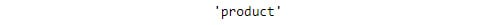##### Figure 1.21: Lemmatized word
`lemmatizer.lemmatize('production')`

With the input "`production`", the following output is generated: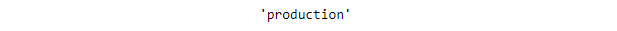##### Figure 1.22: Lemmatized word
`lemmatizer.lemmatize('coming')`

With the input "`coming`", the following output is generated: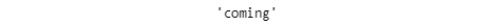##### Figure 1.23: Lemmatized word
`lemmatizer.lemmatize('battle')`

With the input "`battle`", the following output is generated: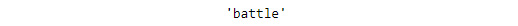##### Figure 1.24: Lemmatized word

We have learned how to use the lemmatization process to transform a given word into its base form.

In the next section, we will look at another preprocessing step in NLP: named entity recognition (NER).

## NER

Named entities are usually not present in dictionaries. So, we need to treat them separately. The main objective of this process is to identify the named entities (such as proper nouns) and map them to the categories that are already defined. For example, the categories might include names of persons, places, and so on. To get a better understanding of this process, we'll look at an exercise.

## Exercise 9: Treating Named Entities

In this exercise, we will find out the named entities in a sentence. Follow these steps to implement this exercise:

1. Open a Jupyter notebook.
2. Insert a new cell and add the following code to import the necessary libraries:
```import nltk
from nltk import word_tokenize
3. Declare the `sentence` variable and assign it a string. Insert a new cell and add the following code to implement this:
`sentence = "We are reading a book published by Packt which is based out of Birmingham."`
4. To find the named entities from the preceding text, insert a new cell and the following code:
```i = nltk.ne_chunk(nltk.pos_tag(word_tokenize(sentence)), binary=True)
[a for a in i if len(a)==1]```

The code generates the following output: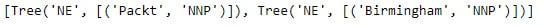##### Figure 1.25: Named entity

In the preceding figure, we can see that the code identifies the named entities "Packt" and "Birmingham" and maps them to an already-defined category such as "NNP".

In the next section, we will focus on word sense disambiguation, which helps us identify the right sense of any word.

## Word Sense Disambiguation

There's a popular saying, "A man is known by the company he keeps". Similarly, a word's meaning depends on its association with other words in the sentence. This means two or more words with the same spelling may have different meanings in different contexts. This often leads to ambiguity. Word sense disambiguation is the process of mapping a word to the correct sense it carries. We need to disambiguate words based on the sense they carry so that they can be treated as different entities when being analyzed. The following figure displays a perfect example of how ambiguity is caused due to the usage of the same word in different sentences:##### Figure 1.26: Word sense disambiguition

To get a better understanding about this process, let's look at an exercise in the next section.

## Exercise 10: Word Sense Disambiguation

In this exercise, we will find the sense of the word "bank" in two different sentences. Follow these steps to implement this exercise:

1. Open a Jupyter notebook.
2. Insert a new cell and add the following code to import the necessary libraries:
```import nltk
from nltk.wsd import lesk
from nltk import word_tokenize```
3. Declare two variables, `sentence1` and `sentence2`, and assign them with appropriate strings. Insert a new cell and the following code to implement this:
```sentence1 = "Keep your savings in the bank"
sentence2 = "It's so risky to drive over the banks of the road"```
4. In order to find the sense of the word "bank" in the preceding two sentences, we make use of the `lesk` algorithm provided by the `nltk.wsd` library. Insert a new cell and add the following code to implement this:
`print(lesk(word_tokenize(sentence1), 'bank'))`

The code generates the following output:##### Figure 1.27: Sense carried by the word "bank" in sentence1

Here, `savings_bank.n.02` refers to a container for keeping money safely at home. To check the other sense of the word bank, write the following code:

`print(lesk(word_tokenize(sentence2), 'bank')) `

The code generates the following output: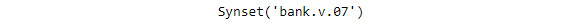##### Figure 1.28: Sense carried by the word "bank" in sentence2

Here, `bank.v.07` refers to a slope in the turn of a road.

Thus, with the help of the `lesk` algorithm, we are able to identify the sense of a word in whatever context. In the next section, we will focus on sentence boundary detection, which helps detect the start and end points of sentences.

## Sentence Boundary Detection

Sentence boundary detection is the method of detecting where one sentence ends and where another sentence begins. If you are thinking that it is pretty easy, as a full stop (.) denotes the end of any sentence and the beginning of another sentence, then you are wrong. This is because there can be instances wherein abbreviations are separated by full stops. Various analyses need to be performed at a sentence level, so detecting boundaries of sentences is essential. An exercise in the next section will provide a better understanding of this process.

## Exercise 11: Sentence Boundary Detection

In this exercise, we will extract sentences from a paragraph. Follow these steps to implement this exercise:

1. Open a Jupyter notebook.
2. Insert a new cell and add the following code to import the necessary libraries:
```import nltk
from nltk.tokenize import sent_tokenize```
3. We make use of the `sent_tokenize()` method to detect sentences in a given text. Insert a new cell and add the following code to implement this:
`sent_tokenize("We are reading a book. Do you know who is the publisher? It is Packt. Packt is based out of Birmingham.")`

The code generates the following output:##### Figure 1.29: List of sentences

As you can see in the figure, we are able to separate out the sentences from given text.

We have covered all the preprocessing steps that are involved in NLP. Now, based on the knowledge we've gained, we will complete an activity in the next section.

## Activity 1: Preprocessing of Raw Text

We have a text corpus that is in an improper format. In this activity, we will perform all the pre-processing steps that were discussed earlier to get some meaning out of the text.

### Note

The `file.txt` file can be found at this location: https://bit.ly/2V3ROAa.

Follow these steps to implement this activity:

1. Import the necessary libraries.
2. Load the text corpus to a variable.
3. Apply the tokenization process to the text corpus and print the first 20 tokens.
4. Apply spelling correction on each token and print the initial 20 corrected tokens as well as the corrected text corpus.
5. Apply PoS tags to each corrected token and print them.
6. Remove stop words from the corrected token list and print the initial 20 tokens.
7. Apply stemming and lemmatization to the corrected token list and the print initial 20 tokens.
8. Detect the sentence boundaries in the given text corpus and print the total number of sentences.

### Note

The solution for this activity can be found on page 254.

By now, you should be familiar with what NLP is and what basic pre-processing steps are needed to carry out any NLP project. In the next section, we will focus on different phases that are included in an NLP project.

# Kick Starting an NLP Project

We can divide an NLP project into several sub-projects or phases. These phases are followed sequentially. This tends to increase the overall efficiency of the process as each phase is generally carried out by specialized resources. An NLP project has to go through six major phases, which are outlined in the following figure: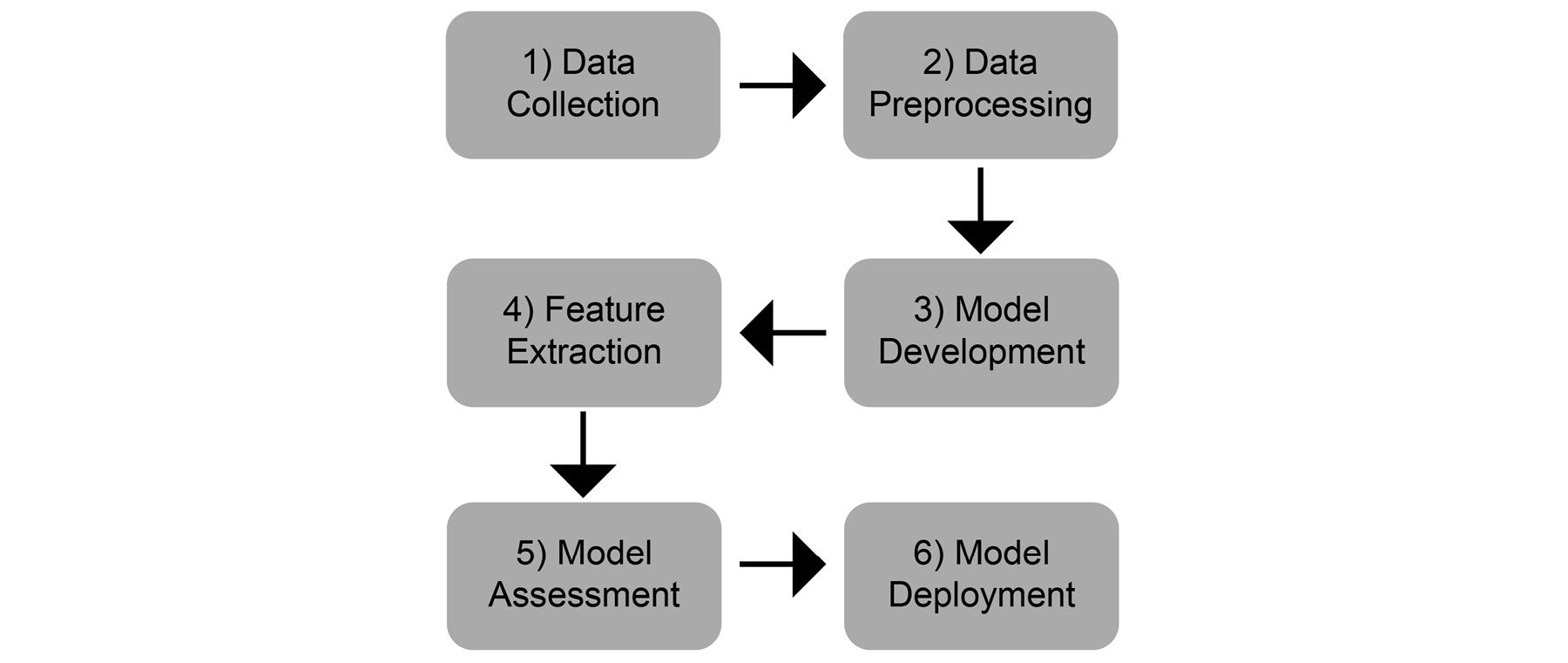##### Figure 1.30: Phases of an NLP project

Suppose you are working on a project in which you need to collect tweets and analyze their sentiments. We will explain how this is carried out by discussing each phase in the coming section.

## Data Collection

This is the initial phase of any NLP project. Our sole purpose is to collect data as per our requirements. For this, we may either use existing data, collect data from various online repositories, or create our own dataset by crawling the web. In our case, we will collect tweets.

## Data Preprocessing

Once the data is collected, we need to clean it. For the process of cleaning, we make use of the different pre-processing steps that we have used in this chapter. It is necessary to clean the collected data, as dirty data tends to reduce effectiveness and accuracy. In our case, we will remove the unnecessary URLs, words, and more from the collected tweets.

## Feature Extraction

Computers understand only binary digits: 0 and 1. Thus, every instruction we feed into a computer gets transformed into binary digits. Similarly, machine learning models tend to understand only numeric data. As such, it becomes necessary to convert the text data into its equivalent numerical form. In our case, we represent the cleaned tweets using different kinds of matrices, such as bag of words and TF-IDF. We will be learning more about these matrices in later chapters.

## Model Development

Once the feature set is ready, we need to develop a suitable model that can be trained to gain knowledge from the data. These models are generally statistical, machine learning-based, deep learning-based, or reinforcement learning-based. In our case, we will build a model that is capable of extracting sentiments from numeric matrices.

## Model Assessment

After developing a model, it is essential to benchmark it. This process of benchmarking is known as model assessment. In this step, we will evaluate the performance of our model by comparing it to others. This can be done by using different parameters or metrics. These parameters include F1, precision, recall, and accuracy. In our case, we will evaluate the newly created model by checking how well it performs when extracting the sentiments of the tweets.

## Model Deployment

This is the final stage for most industrial NLP projects. In this stage, the models are put into production. They are either integrated into an existing system or new products are created by keeping this model as a base. In our case, we will deploy our model to production, such that it can extract sentiments from tweets in real time.

# Summary

In this chapter, we learned how NLP is different from text analytics. We also covered the various pre-processing steps that are included in NLP. We looked at the different phases an NLP project has to pass through. In the next chapter, you will learn about the different methods required for extracting features from unstructured texts, such as TF-IDF and bag of words. You will also learn about NLP tasks such as tokenization, lemmatization, and stemming in more detail. Furthermore, text visualization techniques such as word clouds will be introduced.